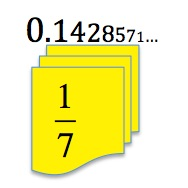Fractions, decimals and percentages are three different ways of writing the same number and it is important that we can convert one form into another.

Divide 2 by 11 to get the decimal fraction equivalent to the common fraction two elevenths $\frac{2}{11}$. Carry on dividing until you see a patttern. Will this pattern continue? Why or why not?

Now use the same method of dividing the numerator by the denominator to find the recurring decimals for all the fractions with 11 as denominator $\frac{1}{11}$$\frac{3}{11}$$\frac{4}{11}$$\frac{5}{11}$$\frac{6}{11}$$\frac{7}{11}$, $\frac{8}{11}$$\frac{9}{11}$ and $\frac{10}{11}$. Do you see a pattern? Can you explain why this pattern occurs?

Divide 1 by 4, then 2 by 4 and then 3 by 4 to get the decimal fractions involving quarters.

Then divide 1 by 5, then 2 by 5, then 3 by 5 and then 4 by 5  to get the decimal fractions involving fifths.

What is the difference between these decimals and the decimals involving elevenths?

Divide 1 by 7 to get the decimal equivalent to the common fraction $\frac{1}{7}$. Carry on dividing until you get 8 places of decimals. Do you see a pattern? Will this pattern continue? Why or why not?

Now do the same to find the recurring decimals for $\frac{2}{7}$$\frac{3}{7}$$\frac{4}{7}$$\frac{5}{7}$ and $\frac{6}{7}$. Do you see a pattern? Can you explain why this pattern occurs?

Tagged with: